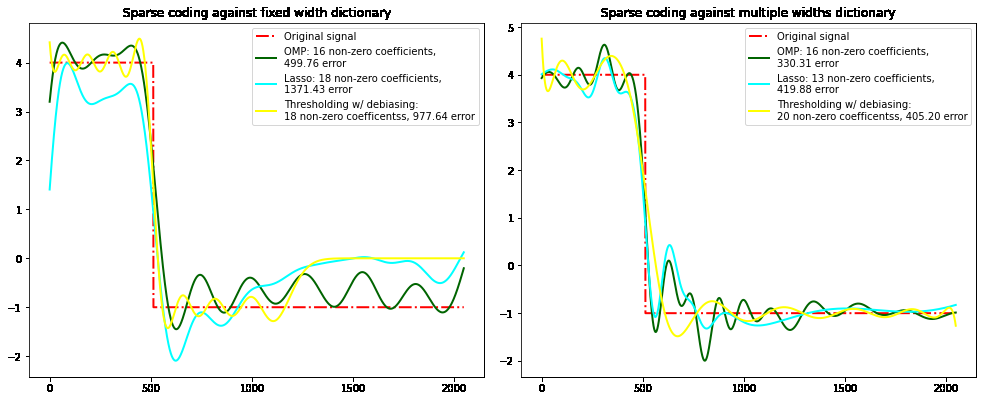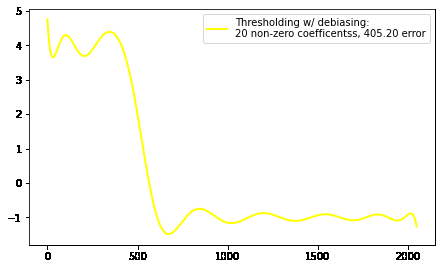Open in App
Not now

# Sparse Coding with a Precomputed Dictionary in Scikit Learn

• Difficulty Level : Expert
• Last Updated : 31 Jan, 2023

A sparse array/matrix is a special type of matrix whose most of the elements are having a value of zero. Generally, the number of non-zero elements in a sparse matrix is equal to the number of rows or columns of the matrix. So, sparse coding can be defined as a representation learning method that is used to find a sparse representation of the input dataset. The representation format is a linear combination of basic elements as well as those basic elements themselves. These elements are called atoms and these atoms compose the dictionary. The elements/atoms in the dictionary may not be orthogonal but rather may be an over-complete spanning set.

Here, we are going to transform a signal into a sparse combination of Ricker dictionary/wavelet. The Ricker(or Mexican Hat) wavelet is defined as the second derivative of the Gaussian function or the third derivative of the normal-probability density function. To implement the sparse coding method we need to know some of its basic parameters and attributes given below.

Parameters:

• transform_algorithm : {‘lasso_lars’, ‘lasso_cd’, ‘lars’, ‘omp’, ‘threshold’}, default=’omp’ :- These algorithms are used for transformation of data. Here we have used lasso_lars and omp.
• lasso_lars : It uses Lars(it uses least angle regression method) to compute the Lasso solution.
• omp : It uses orthogonal matching pursuit to estimate the sparse solution.
• transform_n_nonzero_coefs : int, default=None :- This the total number of non-zero coefficients to target in each column of the solution. This is only used by omp and lars. It is overridden by alpha in the omp algorithm.
• transform_max_iter : int, default=1000 :- The maximum number of iterations to perform in algorithm lasso_lars.
• transform_alpha : float, default=None :- Algorithm lasso_lars  uses alpha as penalty applied to the L1 norm. During threshold computing alpha is the absolute value of the threshold below which coefficients will be squashed to zero. For omp algorithm alpha is the tolerance parameter whose value of the reconstruction error targeted.

Attributes:

• n_features_in_ : int :- The total number of features seen during model fitting.
• n_components_ : int :- It is the total number of elements/atoms.

### Importing libraries and generating sample dataset

By using python libraries like NumPy, Matplotlib, and Sklearn we can easily compute complex computations with a single line of code. After that, we will generate our sample data which will be used later. Here we will mainly define resolution, width, subsampling factor, and the Total number of components used and generate a signal which will be transformed as a sparse combination of Ricker wavelets.

## Python3

 `# importing python libraries``import` `numpy as np``import` `matplotlib.pyplot as plt``from` `sklearn.decomposition ``import` `SparseCoder``# defining sample features``resolution ``=` `2048``subSmlFact ``=` `32``width ``=` `128``noOfComponents ``=` `resolution ``/``/` `subSmlFact``linewidth ``=` `2``estimators ``=` `[``    ``(``"OMP"``, ``"omp"``, ``None``, ``16``, ``"darkgreen"``),``    ``(``"Lasso"``, ``"lasso_lars"``, ``2``, ``None``, ``"cyan"``),``]``# Generate a signal``y ``=` `np.linspace(``0``, resolution ``-` `2``, resolution)``first_quarter ``=` `y < resolution ``/` `4``y[first_quarter] ``=` `4.0``y[np.logical_not(first_quarter)] ``=` `-``1.0`

### Computing ricker Dictionary

For computing the ricker dictionary we need to define the function and a matrix by which we will generate the ricker wavelet dictionary. Here we will generate fixed-width and multiple widths dictionaries.

## Python3

 `# defining ricker function``def` `rickerFunc(resolution, center, width):``    ``x ``=` `np.linspace(``0``, resolution ``-` `1``, resolution)``    ``x ``=` `(``        ``(``2` `/` `(np.sqrt(``3` `*` `width) ``*` `np.pi``*``*``0.25``))``        ``*` `(``1` `-` `(x ``-` `center) ``*``*` `2` `/` `width``*``*``2``)``        ``*` `np.exp(``-``((x ``-` `center) ``*``*` `2``) ``/` `(``2` `*` `width``*``*``2``))``    ``)``    ``return` `x` `# defining ricker matrix``def` `rickerMatrix(width, resolution, n_components):``    ``centers ``=` `np.linspace(``0``, resolution ``-` `1``, n_components)``    ``Dict` `=` `np.empty((n_components, resolution))``    ``for` `i, center ``in` `enumerate``(centers):``        ``Dict``[i] ``=` `rickerFunc(resolution, center, width)``    ``Dict` `/``=` `np.sqrt(np.``sum``(``Dict``*``*``2``, axis``=``1``))[:, np.newaxis]``    ``return` `Dict`  `# Computing wavelet dictionary(fixed-width and multi-widhts)``fixedDict ``=` `rickerMatrix(``    ``width``=``width, resolution``=``resolution, n_components``=``noOfComponents)``multiDict ``=` `np.r_[``    ``tuple``(``        ``rickerMatrix(width``=``w, resolution``=``resolution,``                     ``n_components``=``noOfComponents ``/``/` `5``)``        ``for` `w ``in` `(``10``, ``50``, ``100``, ``500``, ``1000``)``    ``)``]`

### Plotting Results and visualizing the comparison

Here we will define a nested for loop where the 1st for loop will iterate for fixed and multi-width dictionaries and the 2nd/inner for loop will iterate for estimators(OMP, lasso).

## Python3

 `plt.figure(figsize``=``(``14``, ``6``))``# defining nested for-loop``for` `subplot, (``Dict``, title) ``in` `enumerate``(``    ``zip``((fixedDict, multiDict), (``"fixed width"``,``                                 ``"multiple widths"``))``):``    ``plt.subplot(``1``, ``2``, subplot ``+` `1``)``    ``plt.title(``"Sparse coding against %s dictionary"` `%` `title)``    ``plt.plot(y, lw``=``linewidth, color``=``"red"``,``             ``linestyle``=``"-."``,``             ``label``=``"Original signal"``)``    ``# computing a wavelet approximation``    ``for` `title, algo, alpha, n_nonzero,``    ``color ``in` `estimators:``        ``sparseCoder ``=` `SparseCoder(``            ``dictionary``=``Dict``,``            ``transform_n_nonzero_coefs``=``n_nonzero,``            ``transform_alpha``=``alpha,``            ``transform_algorithm``=``algo,``        ``)``        ``x ``=` `sparseCoder.transform(y.reshape(``1``, ``-``1``))``        ``density ``=` `len``(np.flatnonzero(x))``        ``x ``=` `np.ravel(np.dot(x, ``Dict``))``        ``squared_error ``=` `np.``sum``((y ``-` `x) ``*``*` `2``)``        ``plt.plot(``            ``x,``            ``color``=``color,``            ``lw``=``linewidth,``            ``label``=``"%s: %s non-zero coefficients,\n%.2f error"` `%` `(``                ``title, density, squared_error),``        ``)`

Output:After that, we will also use one more transformation algorithm named Threshold and finally visualize the results.

## Python3

 `# Soft thresholding debiasing``sparseCoder ``=` `SparseCoder(``    ``dictionary``=``Dict``,``    ``transform_algorithm``=``"threshold"``,``    ``transform_alpha``=``20``)``x ``=` `sparseCoder.transform(y.reshape(``1``, ``-``1``))``_, idx ``=` `np.where(x !``=` `0``)``x[``0``, idx], _, _, _ ``=` `np.linalg.lstsq(``Dict``[idx, :].T,``                                     ``y, rcond``=``None``)``x ``=` `np.ravel(np.dot(x, ``Dict``))``squared_error ``=` `np.``sum``((y ``-` `x) ``*``*` `2``)``plt.plot(``    ``x,``    ``color``=``"yellow"``,``    ``lw``=``linewidth,``    ``label``=``"Thresholding w``/` `debiasing:\n``%``d non``-``zero\``        ``coefficentss, ``%``.``2f` `error"``    ``%` `(``len``(idx), squared_error),``)``plt.axis(``"tight"``)``plt.legend(shadow``=``False``, loc``=``"best"``)``# adjusting graph view``plt.subplots_adjust(``0.04``, ``0.1``, ``0.98``, ``0.92``, ``0.08``, ``0.2``)``plt.show()`

Output:My Personal Notes arrow_drop_up